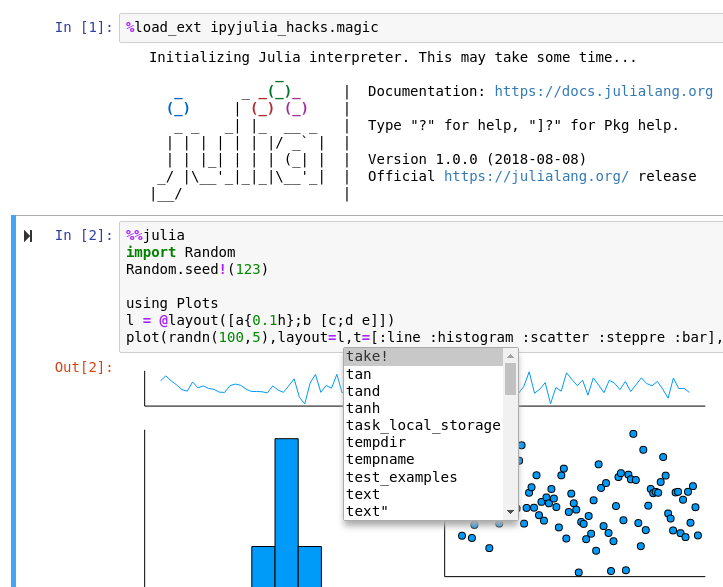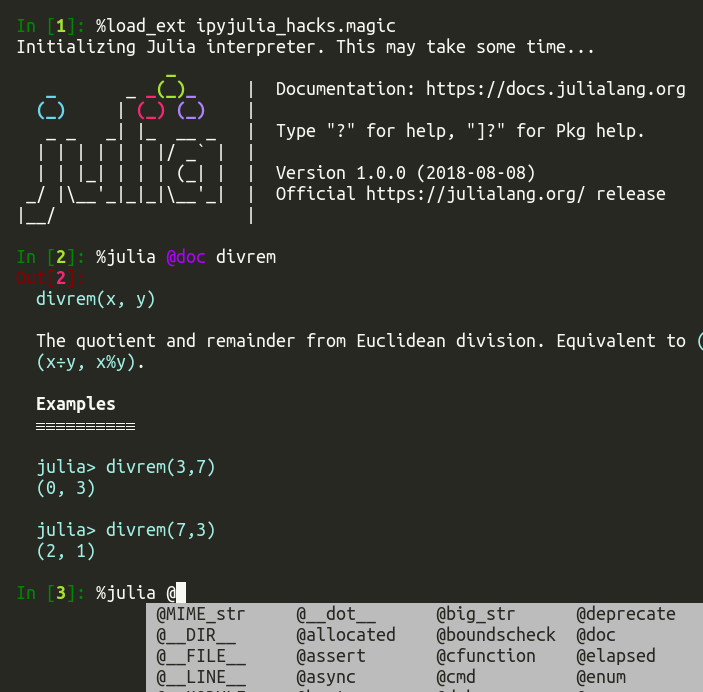# `ipyjulia_hacks`¶

## Horrible hacks for Julia-IPython integration¶

### Screenshots¶

The full notebook for the screenshot below can be found here.### Features¶

• Julia’s Multimedia I/O hooked into IPython’s display system
• Code completion inside Julia magic (by monkey-patching IPython)
• `@async` works in Jupyter (Julia’s event loop is integrated to ipykernel’s asyncio event loop)
• `print` works in Jupyter (Julia’s standard streams are integrated to ipykernel’s I/O)
• Syntax highlighting works in `%%julia` magic of `ipython` CLI (but not in Jupyter)

Those are build on top of the great libraries PyCall.jl and PyJulia. (It would be nice to merge some features to PyJulia at some point. But I wanted to do some experiments on Python interface for handling Julia objects.)

### Installation¶

```pip install https://github.com/tkf/ipyjulia_hacks/archive/master.zip#egg=ipyjulia_hacks
```

#### IPython extension usage¶

```%load_ext ipyjulia_hacks.ipy.magic
```

## API¶

Pythonic wrapper of Julia objects.

```>>> from ipyjulia_hacks import get_api
>>> jlapi = get_api()
```

Mutables:

```>>> spam = jlapi.eval('''Base.eval(Module(), quote
... mutable struct Spam
...     egg
... end
... Spam(1)
... end)''')
>>> spam.egg
1
>>> spam.egg = 2
>>> spam.egg
2
```

Numbers:

```>>> one = jlapi.eval("1", wrap=True)
>>> one
<JuliaObject 1>
>>> one // 2  # translated to ``1 ÷ 2``, *not* ``1 // 2``
0
>>> assert one == 1
>>> assert one != 0
>>> assert one > 0
>>> assert one >= 1
>>> assert one < 2
>>> assert one <= 1
>>> assert one
```

Arrays:

```>>> a2d = jlapi.eval("reshape((1:6) .- 1, (2, 3))")
>>> a2d
<JuliaObject [0 2 4; 1 3 5]>
>>> a2d[0, 1]
2
>>> jlapi.eval("[1, 2, 3]")
array([1, 2, 3], dtype=int64)
>>> arr = jlapi.eval("[1, 2, 3]", wrap=True)
>>> list(reversed(arr))
[3, 2, 1]
```

Linear algebra:

```>>> jlapi.eval("import LinearAlgebra")
>>> I = jlapi.eval("LinearAlgebra.I")
>>> M = jlapi.eval("reshape(1:6, 2, 3)")
>>> Y = M @ I
>>> Y
array([[1, 3, 5],
[2, 4, 6]], dtype=int64)
>>> import numpy
>>> M @ numpy.ones(3)
array([ 9., 12.])
```

Named tuple:

```>>> nt = jlapi.eval("(a = 1, b = 2)")
>>> nt.a
1
>>> nt.b
2
>>> nt
1
>>> nt
2
>>> len(nt)
2
>>> {"a", "b"} <= set(dir(nt))
True
```

Dictionary:

```>>> dct = jlapi.eval('Dict("b" => 2)')
>>> dct["a"] = 1
>>> del dct["b"]
>>> dct["a"]
1
>>> dct
<JuliaObject Dict("a"=>1)>
>>> dct == {"a": 1}
True
```

Three-valued logic:

```>>> true = jlapi.eval("true", wrap=True)
>>> false = jlapi.eval("false", wrap=True)
>>> missing = jlapi.eval("missing")
>>> true
<JuliaObject true>
>>> false
<JuliaObject false>
>>> true & missing
<JuliaObject missing>
>>> false & missing
False
>>> true | missing
True
>>> false | missing
<JuliaObject missing>
>>> true ^ false
True
>>> true ^ true
False
>>> true ^ missing
<JuliaObject missing>
>>> false ^ false
False
```
`ipyjulia_hacks.``get_api`(*args, **kwargs)

Initialize `JuliaAPI`.

Positional and keyword arguments are passed directly to `julia.Julia`

```>>> from ipyjulia_hacks import get_api
>>> get_api(jl_runtime_path="PATH/TO/CUSTOM/JULIA")
<JuliaAPI ...>
```
`ipyjulia_hacks.``get_cached_api`()

Get pre-initialized `JuliaAPI` instance or `None` if not ready.

```>>> from ipyjulia_hacks import get_cached_api
>>> jlapi = get_cached_api()
>>> jlapi.eval("1 + 1")
2
```
class `ipyjulia_hacks.core.``JuliaAPI`(eval_str, api)

Julia-Python interface.

`start_repl`(*, banner=False, history_file=True, **kwargs)

Start Julia REPL.

`eval`(code, wrap=None, **kwargs)

Evaluate `code` in `Main` scope of Julia.

Parameters: Keyword Arguments: code (str) – Julia code to be evaluated. wrap ({True, False, None}) – If `None` (default), wrap the output by a Python interface (`JuliaObject`) for some appropriate Julia objects. Force wrapping by passing None and scope (JuliaObject or PyCall.jlwrap) – A Julia module (default to `Main`).

Examples

```>>> from ipyjulia_hacks import get_api
>>> jlapi = get_api()
```

By default, most of Julia objects returned by this function are the the Python wrapper `JuliaObject`. This object just has a reference to the object held by Julia so that passing it back to Julia is easy. However, you can suppress this behavior by passing `wrap=False`. For example:

```>>> _ = jlapi.eval("dct = Dict()")
>>> dct_jl = jlapi.eval("dct")
>>> dct_py = jlapi.eval("dct", wrap=False)
>>> dct_jl
<JuliaObject Dict{Any,Any}()>
>>> dct_py
{}
>>> assert isinstance(dct_py, dict)
>>> dct_jl["a"] = 1
>>> dct_py["b"] = 2
>>> jlapi.eval("dct")
<JuliaObject Dict{Any,Any}("a"=>1)>
```

Note that `dct` object (living in Julia’s `Main`) does not have the key `"b"`. This is because `dct_py` is a copy of the original Julia object.

Some objects such as `Array` are not wrapped by `JuliaObject` by default. Julia `Array` is automatically converted to `numpy.ndarray` in a copy-free manner by PyCall.jl:

```>>> _ = jlapi.eval("xs = [1, 2, 3]")
>>> xs = jlapi.eval("xs")
>>> xs
array([1, 2, 3], dtype=int64)
>>> xs = 100
>>> jlapi.eval("xs")
array([100,   2,   3], dtype=int64)
```
`getattr`(obj, name)

Get attribute (property) named `name` of a Julia object `obj`.

class `ipyjulia_hacks.core.``JuliaMain`(julia)

An interface to Julia’s `Main` namespace.

`eval`

An alias to `JuliaAPI.eval`.

`import_`(module)

Run `import <module>` in an anonymous module and return `<module>`.

## Demos¶

• Using Plots.jl etc. inside IPython Jupyter kernel: Notebook

### Using ForwardDiff from Python¶

```>>> from ipyjulia_hacks import get_main, jlfunction
>>> @jlfunction
... def f(xs):
...     return sum(xs * 2)
>>> Main = get_main()
>>> ForwardDiff = Main.import_("ForwardDiff")
array([2., 2.])
```

### Fake `asyncio` integration¶

Executable scripts of the following examples can be fond in examples directory.

#### Simple example¶

```async def main():
ajl = AsyncJuliaAPI()
print(await ajl.eval("1"))
try:
await ajl.eval('error("oops!")')
except RuntimeError as err:
print("Expected exception:")
print(err)
else:
raise AssertionError("No exception!")
```

#### Interleaving Python and Julia “background” tasks¶

Suppose you have a Python coroutine that waits for I/O (here just calling `asyncio.sleep`) most of the time:

```async def py_repeat(name, num):
for i in range(num):
print(name, "i =", i)
await asyncio.sleep(0.1)
return f"{name} done"
```

and its Julia equivalent:

```def jl_repeat(ajl, name, num):
return ajl.eval(rf"""
for i in 1:{num}
print("{name} i = \$i\n")
# Using `print` instead of `println` here to force Julia to write
# everything "at once".
sleep(0.1)
end
return "{name} done"
""")
```

Then you can interleave the execution of those tasks in the event loop of `asyncio`:

```async def main():
loop = asyncio.get_event_loop()
ajl = AsyncJuliaAPI()
jl_repeat(ajl, "Julia [A]", 2),
jl_repeat(ajl, "Julia [B]", 5),
py_repeat("Python [A]", 2),
py_repeat("Python [B]", 5),
]))
print(await t)
```

This should output something like:

```Julia [A] i = 1
Julia [B] i = 1
Python [A] i = 0
Python [B] i = 0
Julia [A] i = 2
Julia [B] i = 2
Python [A] i = 1
Python [B] i = 1
Julia [B] i = 3
Python [B] i = 2
Python [B] i = 3
Julia [B] i = 4
Python [B] i = 4
Julia [B] i = 5
Julia [B] done
Python [B] done
Python [A] done
Julia [A] done
```